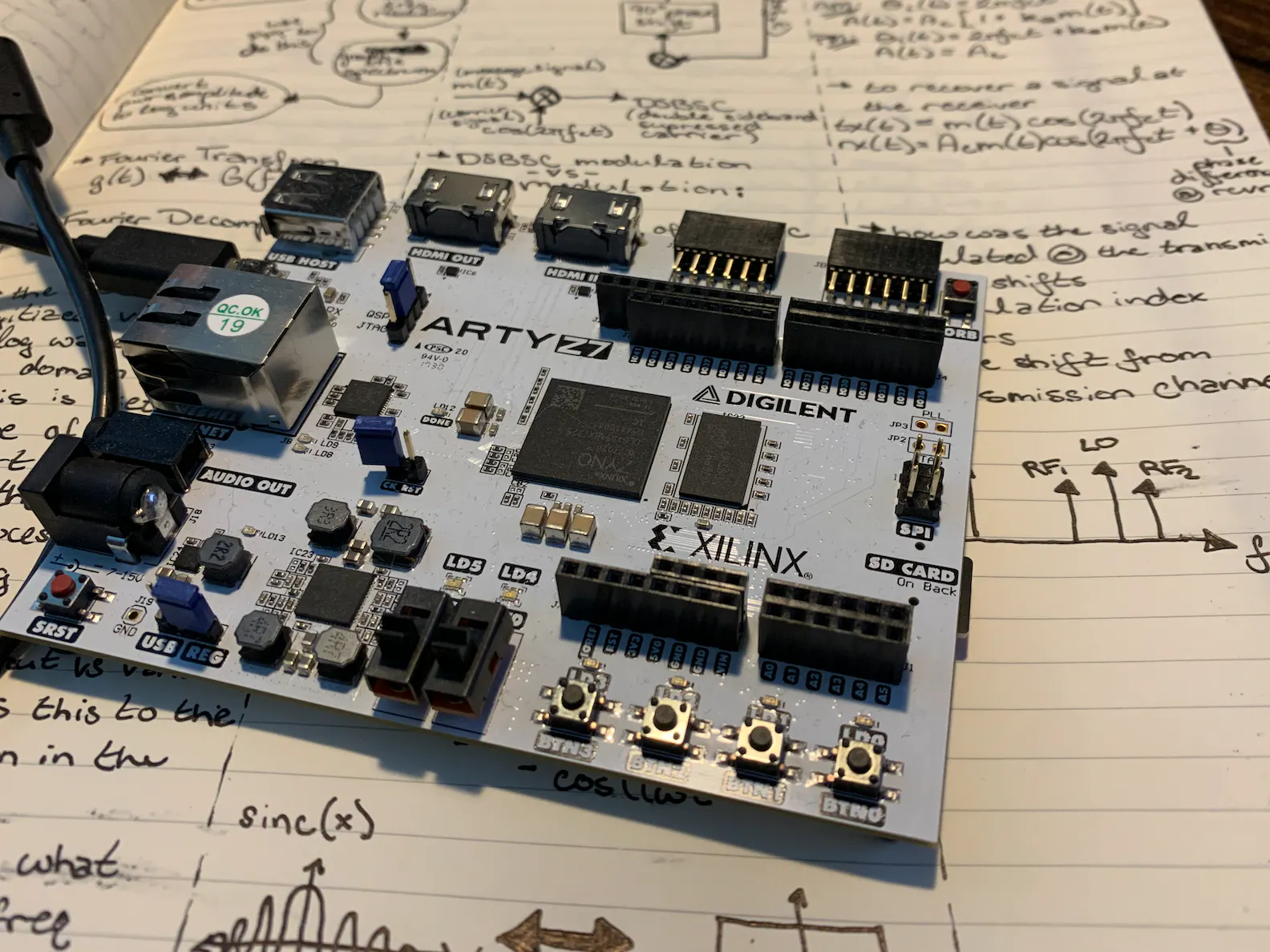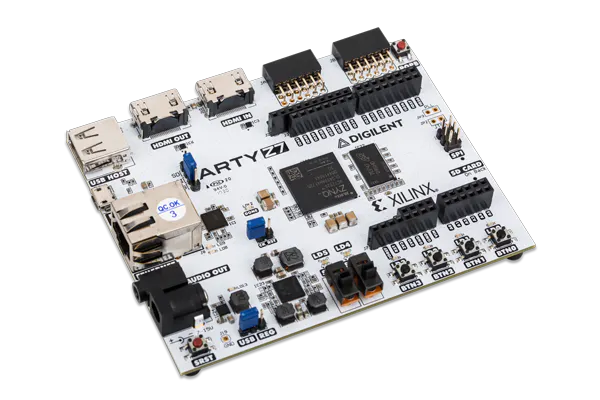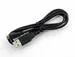# DSP for FPGA: Using Xilinx DDS with Custom FIR

See how to integrate your custom FIR with Xilinx DSP IP such as their DDS Compiler IP.

IntermediateFull instructions provided2 hours3,488## Things used in this project

### Hardware componentsDigilent Arty Z7-20
×1USB-A to Micro-USB Cable
×1

### Software apps and online services## Code

### FIR.v

Verilog
```module FIR(
input clk,
input reset,
input signed [15:0] s_axis_fir_tdata,
input [3:0] s_axis_fir_tkeep,
input s_axis_fir_tlast,
input s_axis_fir_tvalid,
output reg m_axis_fir_tvalid,
output reg m_axis_fir_tlast,
output reg [3:0] m_axis_fir_tkeep,
output reg signed [31:0] m_axis_fir_tdata
);

/* This loop controls the tkeep signal on the master & slave AXI Stream interfaces */
always @ (posedge clk)
begin
m_axis_fir_tkeep <= 4'hf;
end

/* This loop controls the tlast signal on the master & slave AXI Stream interfaces */
always @ (posedge clk)
begin
if (s_axis_fir_tlast == 1'b1)
begin
m_axis_fir_tlast <= 1'b1;
end
else
begin
m_axis_fir_tlast <= 1'b0;
end
end

// 15-tap FIR
reg enable_fir;
reg signed [15:0] buff0, buff1, buff2, buff3, buff4, buff5, buff6, buff7, buff8, buff9, buff10, buff11, buff12, buff13, buff14;
wire signed [15:0] tap0, tap1, tap2, tap3, tap4, tap5, tap6, tap7, tap8, tap9, tap10, tap11, tap12, tap13, tap14;
reg signed [31:0] acc0, acc1, acc2, acc3, acc4, acc5, acc6, acc7, acc8, acc9, acc10, acc11, acc12, acc13, acc14;

/* Taps for LPF running @ 100MSps with passband from 0 Hz - 10 MHz */
assign tap0 = 16'hfe64;
assign tap1 = 16'hfc8a;
assign tap2 = 16'hfc04;
assign tap3 = 16'hff93;
assign tap4 = 16'h0883;
assign tap5 = 16'h14ef;
assign tap6 = 16'h1ff7;
assign tap7 = 16'h2463;
assign tap8 = 16'h1ff7;
assign tap9 = 16'h14ef;
assign tap10 = 16'h0883;
assign tap11 = 16'hff93;
assign tap12 = 16'hfc04;
assign tap13 = 16'hfc8a;
assign tap14 = 16'hfe64;

/* This loop controls the tready & tvalid signals on the master & slave AXI Stream interfaces */
always @ (posedge clk)
begin
if(reset == 1'b0 || m_axis_fir_tready == 1'b0 || s_axis_fir_tvalid == 1'b0)
begin
enable_fir <= 1'b0;
m_axis_fir_tvalid <= 1'b0;
end
else
begin
enable_fir <= 1'b1;
m_axis_fir_tvalid <= 1'b1;
end
end

/* Circular buffer w/ multiply stage of FIR */
always @ (posedge clk)
begin
if(enable_fir == 1'b1)
begin
buff0 <= s_axis_fir_tdata;
acc0 <= tap0 * buff0;

buff1 <= buff0;
acc1 <= tap1 * buff1;

buff2 <= buff1;
acc2 <= tap2 * buff2;

buff3 <= buff2;
acc3 <= tap3 * buff3;

buff4 <= buff3;
acc4 <= tap4 * buff4;

buff5 <= buff4;
acc5 <= tap5 * buff5;

buff6 <= buff5;
acc6 <= tap6 * buff6;

buff7 <= buff6;
acc7 <= tap7 * buff7;

buff8 <= buff7;
acc8 <= tap8 * buff8;

buff9 <= buff8;
acc9 <= tap9 * buff9;

buff10 <= buff9;
acc10 <= tap10 * buff10;

buff11 <= buff10;
acc11 <= tap11 * buff11;

buff12 <= buff11;
acc12 <= tap12 * buff12;

buff13 <= buff12;
acc13 <= tap13 * buff13;

buff14 <= buff13;
acc14 <= tap14 * buff14;
end
end

/* Accumulate stage of FIR */
always @ (posedge clk)
begin
if (enable_fir == 1'b1)
begin
m_axis_fir_tdata <= acc0 + acc1 + acc2 + acc3 + acc4 + acc5 + acc6 + acc7 + acc8 + acc9 + acc10 + acc11 + acc12 + acc13 + acc14;
end
end

endmodule
```

### phase_inc_sm.v

Verilog
```module phase_inc_sm(
input clk,
input reset,
output reg m_axis_phase_tvalid,
output reg m_axis_phase_tlast,
output reg [31:0] m_axis_phase_tdata
);

reg [31:0] carrier_freq;
reg [31:0] carrier_period;

// 1 MHz
wire [31:0] carrier_freq_1m;
wire [31:0] carrier_period_1m;
assign carrier_freq_1m = 32'h51EB85;
assign carrier_period_1m = 32'd100;

// 25 MHz
wire [31:0] carrier_freq_25m;
wire [31:0] carrier_period_25m;
assign carrier_freq_25m = 32'h8000000;

reg [2:0] state_reg;
reg [31:0] period_wait_cnt;

parameter init               = 3'd0;
parameter SetCarrierFreq     = 3'd1;
parameter SetTvalidHigh      = 3'd2;
parameter SetSlavePhaseValue = 3'd3;
parameter WaitState          = 3'd5;
parameter SetTlastHigh       = 3'd6;
parameter SetTlastLow        = 3'd7;

always @ (posedge clk or posedge reset)
begin
// Default Outputs

if (reset == 1'b0)
begin
m_axis_phase_tdata[31:0] <= 32'd0;
state_reg <= init;
end
else
begin
case(state_reg)
init : //0
begin
period_wait_cnt <= 32'd0;
m_axis_phase_tlast <= 1'b0;
m_axis_phase_tvalid <= 1'b0;
carrier_freq <= carrier_freq_1m;
state_reg <= SetCarrierFreq;// WaitForStart;
end

SetCarrierFreq : //1
begin
if (carrier_freq > carrier_freq_25m)
begin
carrier_freq <= carrier_freq_1m;
end
else
begin
carrier_freq <= carrier_freq + carrier_freq_1m;
end

carrier_period <= carrier_period_1m;
state_reg <= SetTvalidHigh;
end

SetTvalidHigh : //2
begin
m_axis_phase_tvalid <= 1'b1; //per PG141 - tvalid is set before tready goes high
state_reg <= SetSlavePhaseValue;
end

SetSlavePhaseValue : //3
begin
m_axis_phase_tdata[31:0] <= carrier_freq;
end

begin
begin
state_reg <= WaitState;
end
else
begin
end
end

WaitState : //5
begin
if (period_wait_cnt >= carrier_period)
begin
period_wait_cnt <= 32'd0;
state_reg <= SetTlastHigh;
end
else
begin
period_wait_cnt <= period_wait_cnt + 1;
state_reg <= WaitState;
end
end

SetTlastHigh : //6
begin
m_axis_phase_tlast <= 1'b1;
state_reg <= SetTlastLow;
end

SetTlastLow : //7
begin
m_axis_phase_tlast <= 1'b0;
state_reg <= SetCarrierFreq;
end

endcase
end
end
endmodule
```

### mem_dump_sm.v

Verilog
```module mem_dump_sm(
input clk,
input reset,
input signed [31:0] s_axis_mem_tdata,
input [3:0] s_axis_mem_tkeep,
input s_axis_mem_tlast,
input s_axis_mem_tvalid,
);

reg signed [31:0] mem_location;

always @ (posedge clk)
begin
if (s_axis_mem_tkeep == 4'hf && s_axis_mem_tvalid == 1'b1)
begin
mem_location <= s_axis_mem_tdata;
end
else
begin
mem_location <= mem_location;
end
end

endmodule
```

## Credits

### Whitney Knitter

80 projects • 926 followers
Working as a full-time SDR/FPGA engineer, but making time for the fun projects at home.